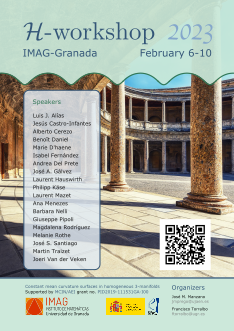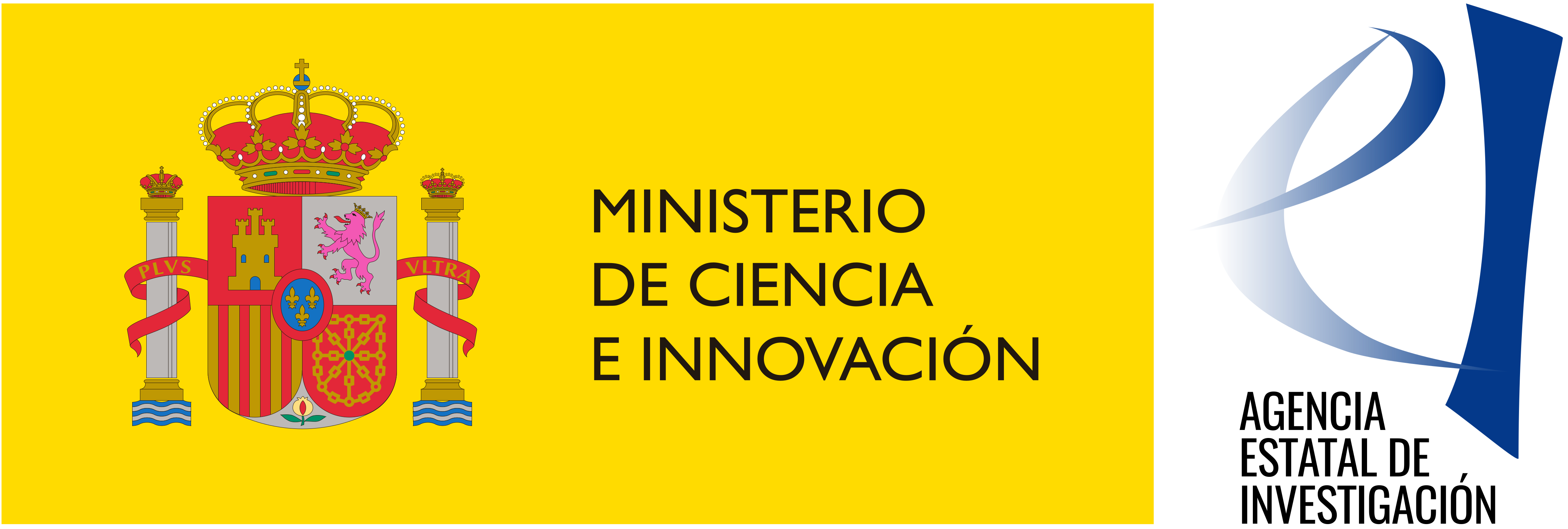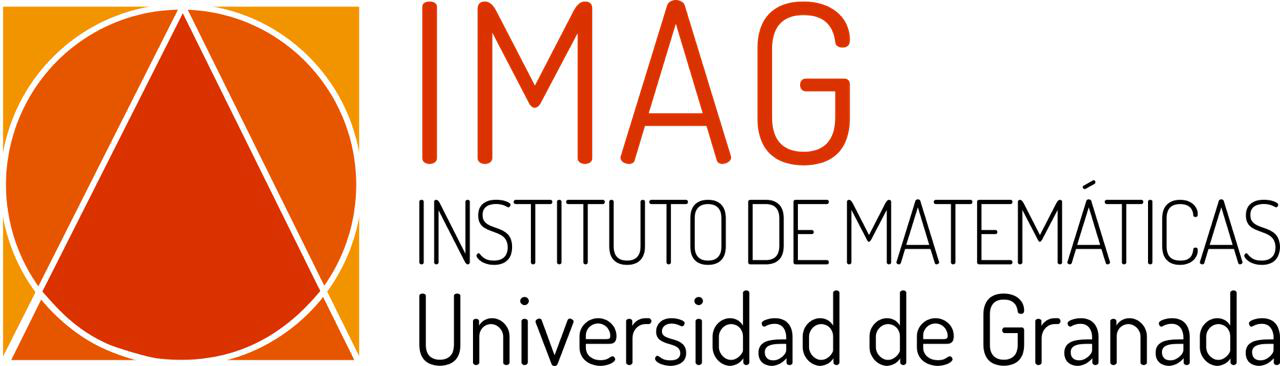## Organizers

José M. Manzano
email:

Francisco Torralbo
email:

## Poster## Supported by

MICIN/AEI grant
PID2019-111531GA-I00Constant mean curvature surfaces and related areas of submanifold theory represent a classical field of research that uses techniques from both Differential Geometry and Geometric Analysis. The aim of this $\mathcal{H}$-workshop is to gather together some distinguished geometers to discuss some cutting-edge discoveries in this field, as well as to give PhD students the opportunity to present their works. This event has been conceived as part of the project constant mean curvature surfaces in homogeneous 3-manifolds, supported by MICIN/AEI grant PID2019-111531GA-I00.

## Registration

Everyone is invited to join the $\mathcal{H}$-workshop 2023. If you would like to attend the conference, please email us to or , so that we can estimate the number of participants.

## Programme

Participants are invited to arrive in IMAG on Monday 6th to visit the facilities and meet the staff at the University of Granada. Some spaces have been reserved to foster discussion and collaborations between participants and/or local researchers on Friday 10th. Please, contact us if you want to use these spaces so we can allocate you properly!

Tue 7 Wed 8 Thu 9
9:00 opening Traizet Traizet
9:30 Hauswirth
10:00 Pipoli D'haene
coffee coffee coffee
11:00 Menezes Nelli Van der Veken
11:30
12:00 Rothe Daniel Alías
12:30 Santiago

15:30 Del Prete Käse Fernández
16:00 Cerezo Castro-Infantes
16:30 Mazet Rodríguez Gálvez
17:00

20:30   dinner
abstract pdf Luis J. Alías Universidad de Murcia Spacelike submanifolds into the light cone of the Lorentz-Minkowski spacetime
Abstract: In this lecture we consider codimension two spacelike submanifolds contained in the light cone of the Lorentz-Minkowski spacetime using an approach which allows us to compute their extrinsic and intrinsic geometries in terms of a single function $u$. As a first application of our approach, we classify the totally umbilical ones. For codimension two compact spacelike submanifolds into the light cone, we show that they are conformally diffeomorphic to the round sphere and that they are given by an explicit embedding written in terms of $u$. We also consider the case where the submanifold is (marginally, weakly) trapped. In particular, we derive some non-existence results for weakly trapped submanifolds into the light cone. This is a joint work with Verónica L. Cánovas and Marco Rigoli.
abstract Jesús Castro-Infantes IMAG - Universidad de Granada A construction of genus one $H$-surfaces in $\mathbb{H}^2\times\mathbb{R}$
Abstract: In this talk we will construct via Daniel's sister correspondence in $\mathbb H^2\times\mathbb R$ two families of properly Alexandrov-immersed surfaces with constant mean curvature $0\lt H\lt 1/2$ in $\mathbb H^2\times \mathbb R$ with genus one and $k$ ends asymptotic to vertical $H$-cylinder. Moreover, we will show the existence of properly Alexandrov-immersed $H$ surfaces with genus one and $2$ ends each of them asymptotic to a vertical cylinder from the convex side displaying that there is not a Schoen-type theorem for immersed $H$-surfaces in $\mathbb H^2\times\mathbb R$. This is a joint work with J. Santiago.
abstract pdf arxiv Alberto Cerezo IMUS - Universidad de Sevilla Embedded free boundary CMC annuli in the unit ball
Abstract: Wente (1995) constructed the first known examples of non-rotational, free boundary annuli with constant mean curvature $H\neq 0$ in the unit ball $\mathbb{B}^3 \subset \mathbb{R}^3$. These annular surfaces were not embedded.

With respect to the case $H = 0$, Fernández, Hauswirth and Mira have recently obtained examples of free boundary minimal annuli in $\mathbb{B}^3$.

In this talk, we will discuss the construction of a one-parameter family of embedded CMC annuli with free boundary in $\mathbb{B}^3$ and a prismatic symmetry group of order $4n$ for any $n\gt 1$. They constitute the first known examples of embedded non-minimal CMC annuli with free boundary in the unit ball apart from Delaunay surfaces, and the first annular solutions to the partitioning problem in the ball that are not rotational. This is a joint work with Isabel Fernández and Pablo Mira.
abstract pdf Benoît Daniel Institut Élie Cartan (Lorraine) On constant mean curvature surfaces in product manifolds
Abstract: In this talk we will classify constant mean curvature surfaces with constant intrinsic curvature in the product manifolds $\mathbb{S}^2\times\mathbb{R}$ and $\mathbb{H}^2\times\mathbb{R}$, where $\mathbb{S}^2$ is the constant curvature sphere and $\mathbb{H}^2$ the hyperbolic plane. We will also consider parallel mean curvature surfaces with constant intrinsic curvature in the product manifolds $\mathbb{S}^2\times\mathbb{S}^2$ and $\mathbb{H}^2\times\mathbb{H}^2$. In particular these classifications will provide new examples. This is a joint work with I. Domingos and F. Vitório.
abstract Marie D'haene KU Leuven Submanifolds of the four-dimensional Thurston geometry $\mathrm{Sol}_0^4$
Abstract: The structure of the talk is as follows: first we will spend some time motivating the importance of Thurston geometries. The main idea is that Thurston geometries are models for a large class of manifolds in dimension two and three (cf. the uniformization of surfaces and the geometrization of three-manifolds). Then, we will focus on one particular four-dimensional Thurston geometry: $\mathrm{Sol}_0^4$, a solvable Lie group.

I will discuss the classification of hypersurfaces in $\mathrm{Sol}_0^4$ admitting a second fundamental form which is a Codazzi tensor (work which was supervised by J. Van der Veken). In particular, these include totally geodesic hypersurfaces. The existence of totally geodesic hypersurfaces in a homogeneous space puts severe restrictions on the ambient space, as a theorem of Nikolayevsky shows. Hence, as a nice corollary of the aforementioned classification we obtain the possible representations of $\mathrm{Sol}_0^4$ as a warped product. In addition, I will discuss the classification of totally geodesic surfaces in $\mathrm{Sol}_0^4$ (joint work with A. Rodríguez and supervised by J. Van der Veken).

The methods used to obtain these classifications include exploiting the Codazzi equation and making use of a theorem by E. Cartan which gives a correspondence between totally geodesic submanifolds and curvature-invariant subspaces of the tangent space.
abstract pdf arxiv Isabel Fernández IMUS - Universidad de Sevilla Bernstein type results for elliptic Weingarten surfaces
Abstract: A surface in Euclidean 3-space is an elliptic Weingarten surface if its mean curvature H and Gaussian curvature $K$ are related by a smooth, elliptic equation $W(H,K)=0$. A well known open problem, proposed for instance by Rosenberg and Sa Earp in 1994, is to solve the Bernstein problem for this class of surfaces, that is: are planes the only entire elliptic Weingarten graphs? Up to now, it is only known that the answer is positive if the Weingarten equation is uniformly elliptic; this follows from a deep theorem by L. Simon on entire graphs with quasiconformal Gauss map. In this talk we present two theorems. In the first one, we extend the solution to the Bernstein problem in the uniformly elliptic case to multigraphs, proving that planes are the only complete uniformly elliptic Weingarten surfaces whose Gauss map image lies in an open hemisphere. In the second one, we will solve in the affirmative the Bernstein problem for Weingarten graphs for a large class of non-uniformly elliptic Weingarten equations. This is a joint work with Pablo Mira and José A. Gálvez.
abstract pdf Andrea Del Prete Univ. degli Studi dell'Aquila - Univ. de Jaén A Twin Correspondence for Surfaces in Killing submersions
Abstract: A Killing submersion is a (semi-)Riemannian submersion $\pi\colon\mathbb{E}\to M$, where $\mathbb{E}$ and $M$ are orientable and connected, such that the fibers of $\pi$ are the integral curves of a nowhere vanishing (temporal) Killing vector field $\xi$ on the three-manifold $\mathbb{E}$. In this setting, we give a suitable definition of the graph of a function $f\in \mathcal{C}^\infty(U)$, where $U$ is an open subset of $M$, and we prove a generalized Calabi-type duality between (spacelike) graphs of prescribed mean curvature in Riemannian and semi-Riemannian Killing submersions. Finally we use this result to prove the existence of entire spacelike graph of certain prescribed mean curvature in Lorentz-Minkowski space $\mathbb{L}^3$. This is a joint work with J. M. Manzano.
abstract José A. Gálvez IMAG - Universidad de Granada The index of the Hessian of an analytic saddle graph and immersed spheres
Abstract: We will show that every analytic sphere immersed in $S^3$, with principal curvatures $k_1,k_2$ fulfilling $k_1 k_2\leq 0$ must be totally umbilical. This extends well-known results from Alexandrov or Almgren among others. To do this, we will prove a result on analytic saddle graphs that show that the index theory can be used on an analytical surface satisfying the previous inequality even when the set of umbilical points is not discrete. This is in sharp contrast to the existence of non totally umbilical smooth spheres satisfying $k_1k_2\leq 0$. It is a joint work with Pablo Mira and Marcos Tassi.
abstract Laurent Hauswirth Université de Marne-la-Vallée Minimal annuli and Spectral curves
Abstract: No abstract.
abstract Philipp Käse TU Darmstadt New CMC tubes in homogeneous spaces
Abstract: In 1841 Delaunay characterised the surfaces of constant mean curvature $H=1$ in Euclidean 3-space invariant under rotation. The result was generalized by several authors to screw-motion invariance on the one hand and to other ambient homogeneous 3-manifolds on the other hand. In this talk I want to show, that in this general case a new surface type can arise in the family of Delaunay surfaces: CMC tubes. I will present conditions for existence and non-existence.
abstract Laurent Mazet Institut Denis Poisson (Tours) Free boundary minimal surfaces outside of the ball
Abstract: Free boundary minimal surfaces in a domain $\Omega$ are minimal surfaces that intersect orthogonally the boundary $\partial\Omega$. In this talk I will consider the case where $\Omega$ is the complement of the unit ball in $\mathbb{R}^n$. I will describe the rotationally invariant examples and give some classification results. This is a joint work with Abraão Mendes.
abstract Ana Menezes Princeton University A two-piece property for free boundary minimal surfaces in the ball
Abstract: In this talk we will prove that every plane passing through the origin divides an embedded compact free boundary minimal surface of the euclidean 3-ball in exactly two connected surfaces. This is the analog of a well-known result by Antonio Ros in the sphere $\mathbb{S}^3$; and our result gives evidence to a conjecture by Ailana Fraser and Martin Li. This is a joint work with Vanderson Lima from UFRGS, Brazil.
abstract pdf Barbara Nelli Università degli Studi dell'Aquila Hypersurfaces with constant higher order mean curvature
Abstract: We discuss some old and more recent results about the shape of a hypersurface with constant higer order mean curvature.
abstract Giuseppe Pipoli Università degli Studi dell'Aquila Constant mean curvature hypersurfaces in $\mathbb H^n\times\mathbb R$ with small planar boundary
Abstract: We show that constant mean curvature hypersurfaces in $\mathbb H^n\times\mathbb R$ with small boundary contained in a horizontal slice $P$ are topological disks, provided they are contained in one of the two halfspaces determined by $P$. This is the analogous in $\mathbb H^n\times\mathbb R$ of a result in $\mathbb R^3$ by A. Ros and H. Rosenberg. This talk is based on a joint work with Barbara Nelli.
abstract Magdalena Rodríguez IMAG - Universidad de Granada Minimal surfaces with finite total curvature in $\mathbb{H}^2\times\mathbb{R}$
Abstract: I will give an overview of the theory of minimal surfaces with finite total curvature in the product space of the hyperbolic plane and the real line. I will describe the geometry of the ends of one such surface and show some known and new examples. Finally I will present a characterization theorem for such surfaces and some classification results.
abstract pdf arxiv Melanie Rothe TU Darmstadt Lawson's Bipolar Minimal Surfaces in the 5-Sphere
Abstract: Unlike Euclidean space $\mathbb{R}^n$, the n-sphere $\mathbb{S}^n$ allows for closed minimal surfaces. Interestingly, the latter turned out to be highly relevant in the geometric optimization of Laplacian eigenvalues or the Willmore energy under fixed topology. However, regarding each topological class, the list of known examples is very limited. Concerning higher codimensions, H. B. Lawson has shown in 1970 that every minimal surface in $\mathbb{S}^3$ induces a minimal surface in $\mathbb{S}^5$, its so-called bipolar surface. For closed surfaces, the example of the bipolar Lawson surfaces $\widetilde{\tau}_{m,k}\subseteq\mathbb{S}^5$ showed that the topology can thereby undergo a change. From this perspective, we give a topological classification of the bipolar Lawson surfaces $\tilde{\xi}_{m,k}$ and $\tilde{\eta}_{m,k}$. Additionally, we provide upper and lower area bounds, and find that these surfaces are not embedded for $m\geq 2$ or $k\geq 2$.
abstract José S. Santiago Universidad de Jaén Fundamental data for surfaces in homogenous Lorentzian 3-manifolds
Abstract: In this talk we will discuss an analogue to Daniel’s correspondence on the 3-dimensional Lorentzian manifolds $\mathbb{L}(\kappa,\tau)$ with isometry group of dimension 4. We define some compatibility equations on a surface and prove that they give sufficient conditions for the surface to be immersed in $\mathbb{L}(\kappa,\tau)$ as a spacelike surface. As a consequence, we obtain a Lorentzian correspondence over these spaces for spacelike CMC surfaces. Moreover, under the same perspective, we describe Lee’s correspondence in terms of these compatibility equations and show that it commutes with the Daniel-type correspondences. This is a joint work with I. Castro and J.M. Manzano.
abstract pdf Martin Traizet Institut Denis Poisson (Tours) The DPW method for minimal surfaces in the 3-sphere
The DPW method, also called generalized Weierstrass representation, is a way to represent CMC surfaces (or more generally, harmonic map from a surface to a symmetric space) in term of holomorphic data. In this series of talks, I will focus on minimal surfaces in the 3-sphere.

In the first talk, I will explain the basics of the DPW method (Loop groups, Sym-Bobenko formula, gauges, monodromy problem) and I will present basic examples.

In the second talk, I will present a more advanced example: Lawson minimal surfaces in the 3-sphere. The approach yields fine estimates for their area. Suprisingly, the asymptotic expansion of the area when the genus goes to infinity involves values of the Rieman zeta function. (Joint work with Lynn Heller, Sebastian Heller and Steven Charlton.)
abstract Joeri Van der Veken KU Leuven Minimal Lagrangian submanifolds of complex (hyperbolic) quadrics
Abstract: The complex quadric and its non-compact dual, the complex hyperbolic quadric, are Kähler-Einstein spaces that exist in any complex dimension. In this work, we study minimal Lagrangian submanifolds of these spaces and give classifications under additional assumptions. A key observation is that these Lagrangian immersions can be seen as Gauss maps of hypersurfaces of spheres and of spacelike hypersurfaces of anti-de Sitter spaces respectively. All this can be seen as a generalization of the work of I. Castro and F. Urbano on minimal Lagrangian surfaces in $\mathbb{S}^2\times\mathbb{S}^2$ [Comm. Anal. Geom. 15 (2007), 217–248], since this ambient space is precisely the complex two-dimensional quadric.

## How to get to IMAG

The Maths Institute is located next to the Faculty of Social Sciences of the University of Granada. To get there, you have to access via the Faculty of Social Sciences or via the Documentation Center, both located in the street Rector López Argüeta.

2022-23 © J.M. Manzano - F. Torralbo
This website uses free software.